CSE-4/562 Spring 2021 - Indexing

March 2, 2021

### Today

Leveraging Organization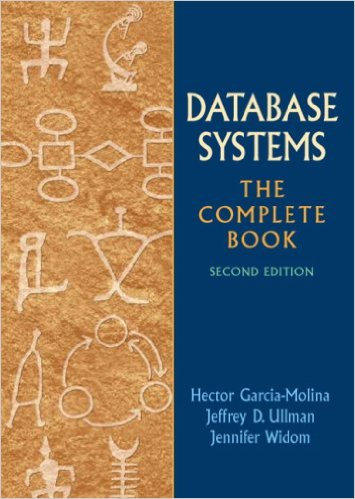$150$50 IndexToC No IndexToC Summary

### Today's Focus

$\sigma_C(R)$ and $(\ldots \bowtie_C R)$

(Finding records in a table really fast)$\sigma_{R.A = 7}(R)$

Where is the data for key 7?

Option 1: Linear search

$O(N)$ IOs

### Initial Assumptions

Data is sorted on an attribute of interest (R.A)

Option 2: Binary Search

$O(\log_2 N)$ IOs

Better, but still not ideal.

Idea: Precompute several layers of the decision tree and store them together.

### Fence Pointers

... but what if we need more than one page?

### ISAM Trees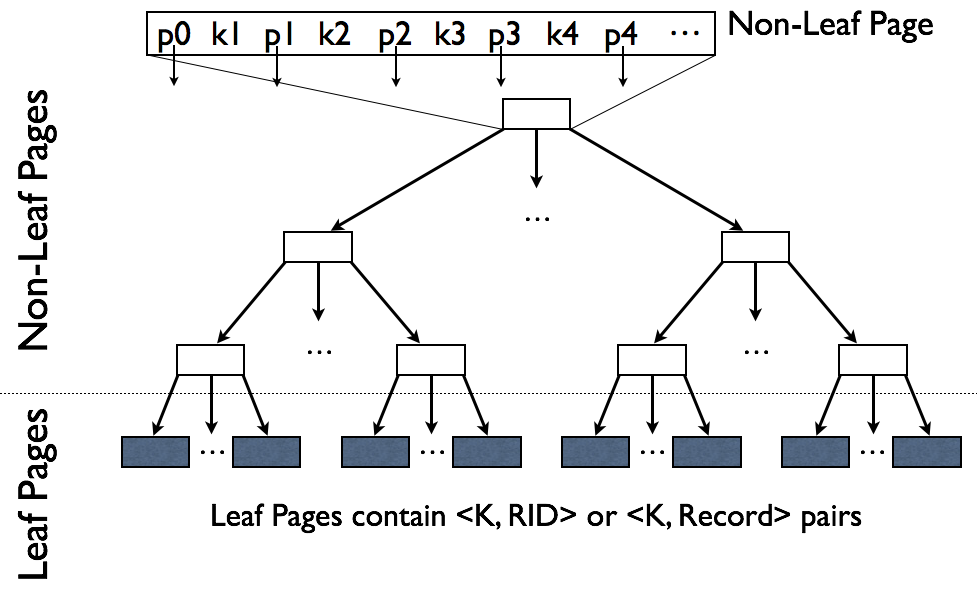Which of the following is better?### Worst-Case Tree?

$O(N)$ with the tree laid out left/right-deep

### Best-Case Tree?

$O(\log N)$ with the tree perfectly balanced

It's important that the trees be balanced

... but what if we need to update the tree?

### Challenges

• Finding space for new records
• Keeping the tree balanced as new records are added

Idea 1: Reserve space for new records

Just maintaining open space won't work forever...

### Rules of B+Trees

Keep space open for insertions in inner/data nodes.
‘Split’ nodes when they’re full
Avoid under-using space
‘Merge’ nodes when they’re under-filled

Maintain Invariant: All Nodes ≥ 50% Full

(Exception: The Root)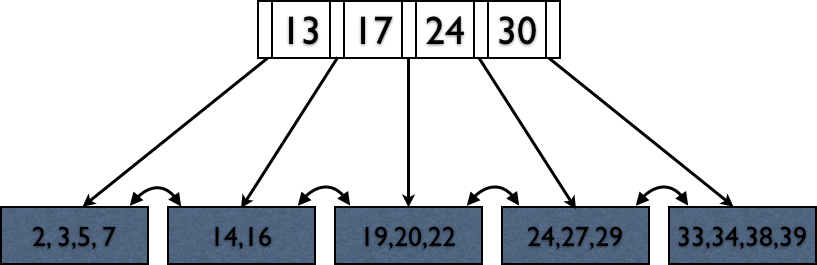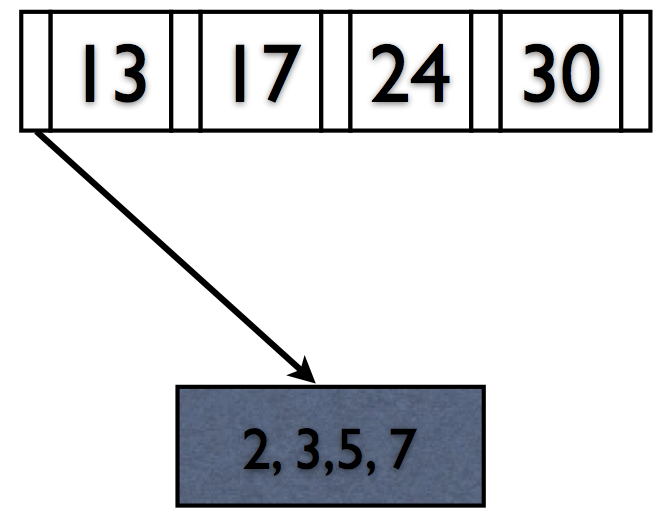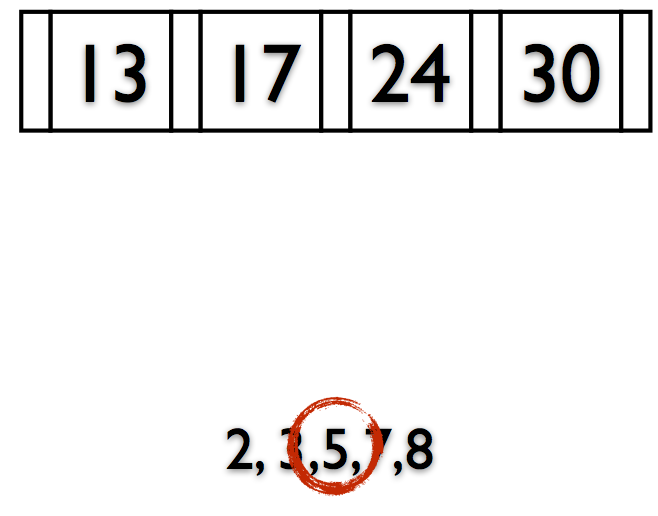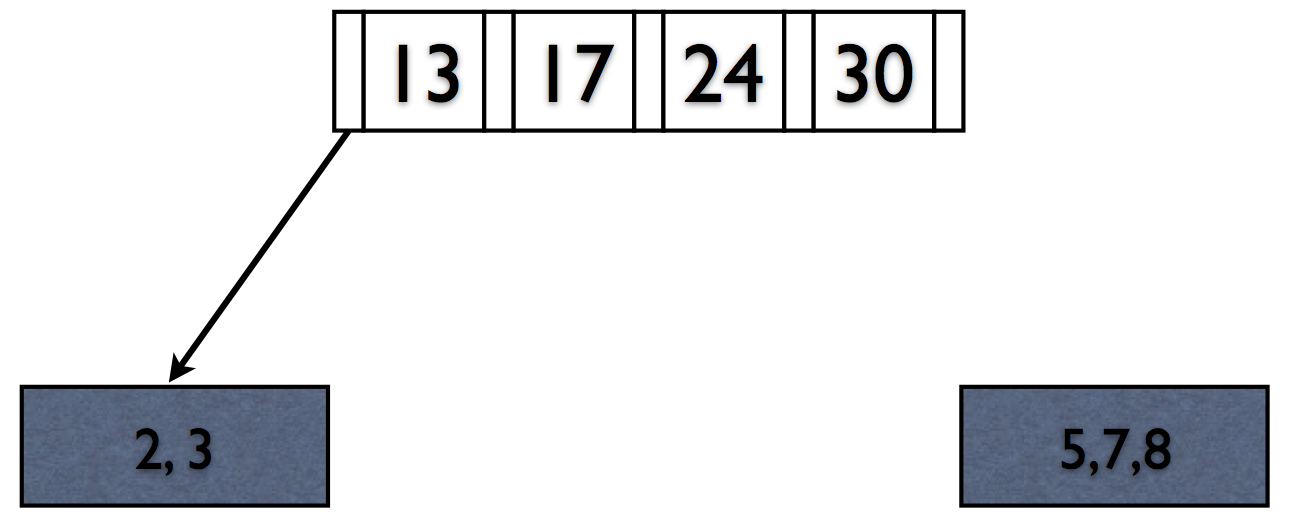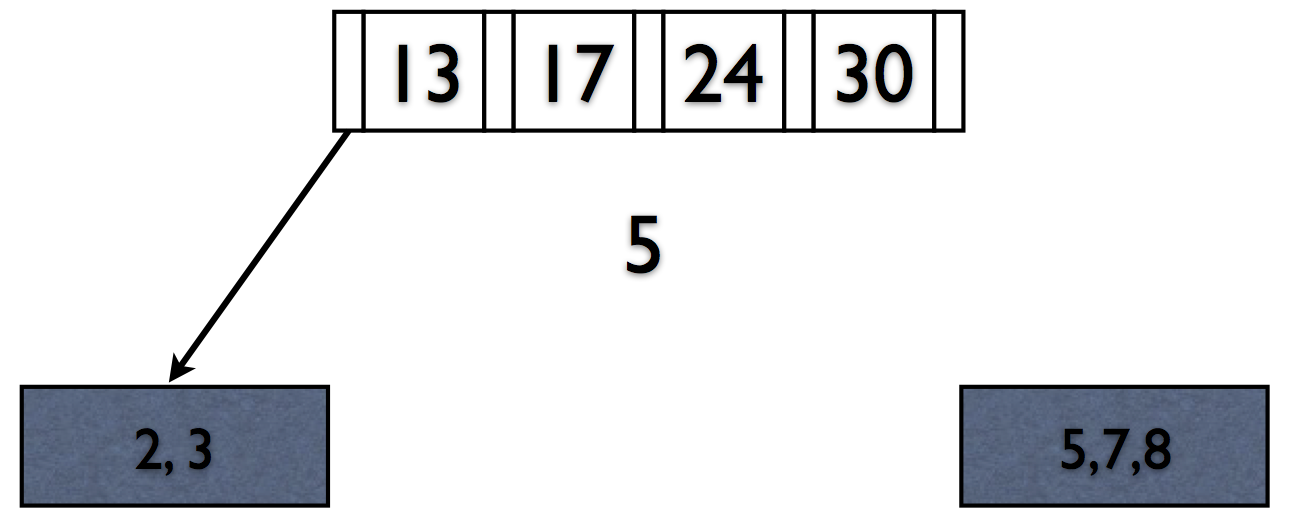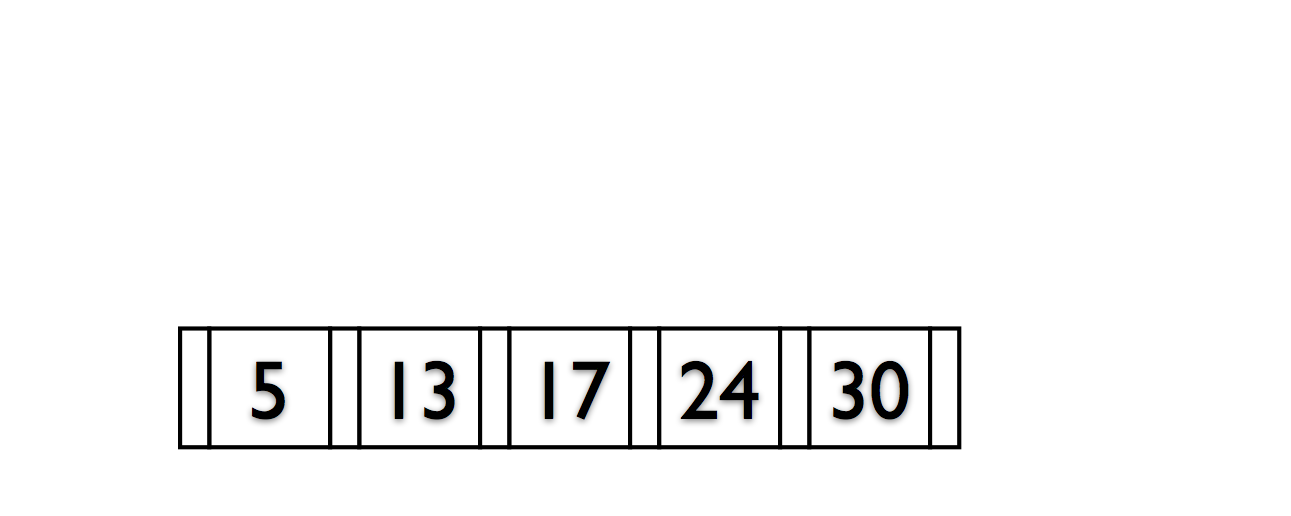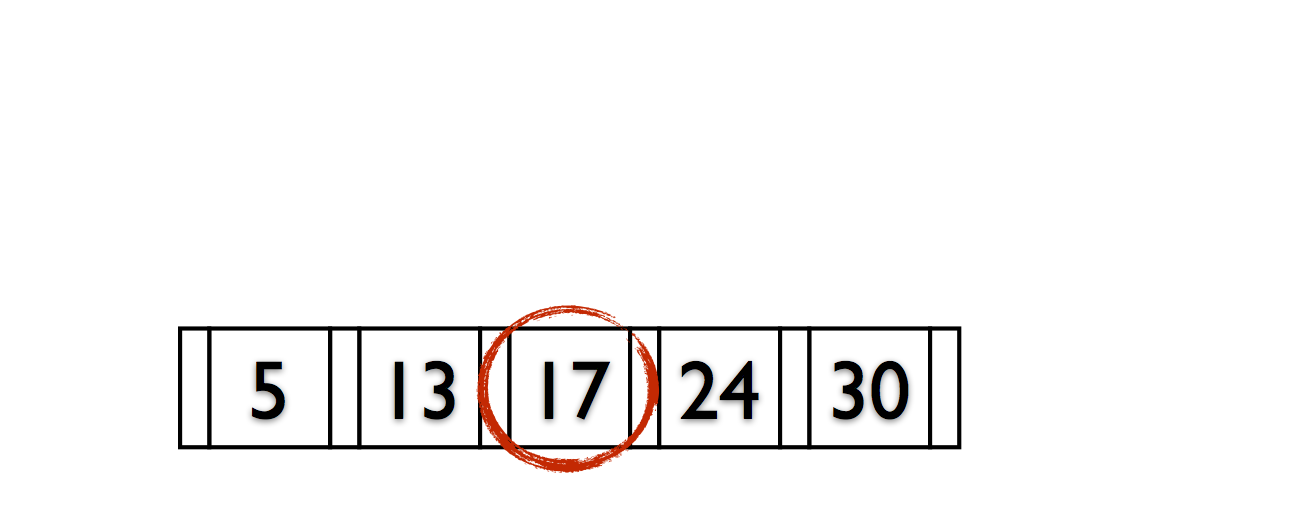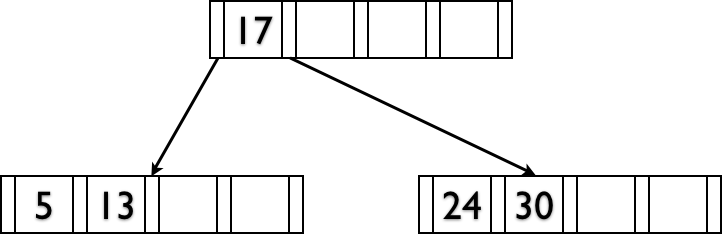Deletions reverse this process (at 50% fill).

### Incorporating Trees into Queries

$\sigma_C(R)$ and $(\ldots \bowtie_C R)$

Original Query: $\pi_A\left(\sigma_{B = 1 \wedge C < 3}(R)\right)$

Possible Implementations:

$\pi_A\left(\sigma_{B = 1 \wedge C < 3}(R)\right)$
Always works... but slow
$\pi_A\left(\sigma_{B = 1}( IndexScan(R,\;C < 3) ) \right)$
Requires a non-hash index on $C$
$\pi_A\left(\sigma_{C < 3}( IndexScan(R,\;B=1) ) \right)$
Requires a any index on $B$
$\pi_A\left( IndexScan(R,\;B = 1, C < 3) \right)$
Requires any index on $(B, C)$

### Lexical Sort (Non-Hash Only)

Sort data on $(A, B, C, \ldots)$

First sort on $A$, $B$ is a tiebreaker for $A$,
$C$ is a tiebreaker for $B$, etc...

All of the $A$ values are adjacent.
Supports $\sigma_{A = a}$ or $\sigma_{A \geq b}$
For a specific $A$, all of the $B$ values are adjacent
Supports $\sigma_{A = a \wedge B = b}$ or $\sigma_{A = a \wedge B \geq b}$
For a specific $(A,B)$, all of the $C$ values are adjacent
Supports $\sigma_{A = a \wedge B = b \wedge C = c}$ or $\sigma_{A = a \wedge B = b \wedge C \geq c}$
...

### For a query $\sigma_{c_1 \wedge \ldots \wedge c_N}(R)$

1. For every $c_i \equiv (A = a)$: Do you have any index on $A$?
2. For every $c_i \in \{\; (A \geq a), (A > a), (A \leq a), (A < a)\;\}$: Do you have a tree index on $A$?
3. For every $c_i, c_j$, do you have an appropriate index?
4. A simple table scan is also an option

Which one do we pick?

(You need to know the cost of each plan)

These are called "Access Paths"

### Strategies for Implementing $(\ldots \bowtie_{c} S)$

Sort/Merge Join
Sort all of the data upfront, then scan over both sides.
In-Memory Index Join (1-pass Hash; Hash Join)
Build an in-memory index on one table, scan the other.
Partition Join (2-pass Hash; External Hash Join)
Partition both sides so that tuples don't join across partitions.
Index Nested Loop Join
Use an existing index instead of building one.

### Index Nested Loop Join

To compute $R \bowtie_{S.B > R.A} S$ with an index on $S.B$
1. Read one row of $R$
2. Get the value of $a = R.A$
3. Start index scan on $S.B > a$
4. Return all rows from the index scan
5. Read the next row of $R$ and repeat

### Index Nested Loop Join

To compute $R \bowtie_{S.B\;[\theta]\;R.A} S$ with an index on $S.B$
1. Read one row of $R$
2. Get the value of $a = R.A$
3. Start index scan on $S.B\;[\theta]\;a$
4. Return all rows from the index scan
5. Read the next row of $R$ and repeat

What if we need multiple sort orders?

### Data Organization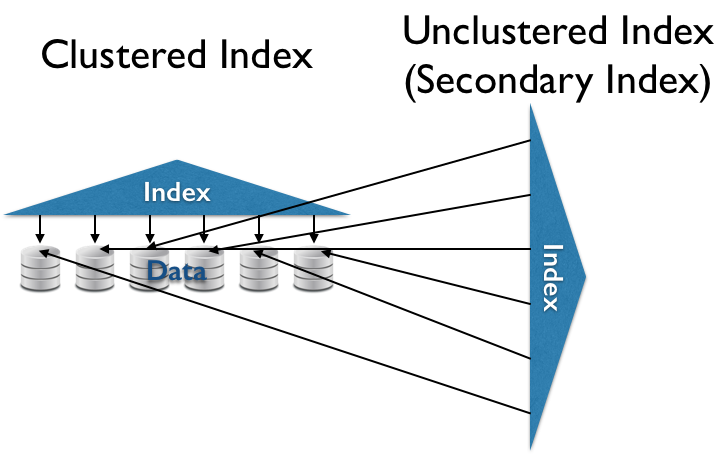### Data Organization### Data Organization

Unordered Heap
$O(N)$ reads.
Sorted List
$O(\log_2 N)$ random reads for some queries.
Clustered (Primary) Index
$O(\ll N)$ sequential reads for some queries.
(Secondary) Index
$O(\ll N)$ random reads for some queries.

### Hash Indexes

A hash function $h(k)$ is ...

... deterministic
The same $k$ always produces the same hash value.
... (pseudo-)random
Different $k$s are unlikely to have the same hash value.

$h(k)\mod N$ gives you a random number in $[0, N)$

### Problems

$N$ is too small
Too many overflow pages (slower reads).
$N$ is too big
Too many normal pages (wasted space).

Idea: Resize the structure as needed

To keep things simple, let's use $$h(k) = k$$

(you wouldn't actually do this in practice)

Changing hash functions reallocates everything randomly

Need to keep the entire source and hash table in memory!

$$h(k) \mod N$$ vs $$h(h) \mod 2N$$

if $h(k) = x \mod N$

then

$h(k) =$ either $x$ or $2x \mod 2N$

Each key is moved (or not) to precisely one of two buckets in the resized hash table.

Never need more than 3 pages in memory at once.

Changing sizes still requires reading everything!

Idea: Only redistribute buckets that are too big

Add a directory (a level of indirection)

• $N$ hash buckets = $N$ directory entries
(but $\leq N$ actual pages)
• Directory entries point to actual pages on disk.
• Multiple directory entries can point to the same page.
• When a page fills up, it (and its directory entries) split.

### Dynamic Hashing

• Add a level of indirection (Directory).
• A data page $i$ can store data with $h(k)%2^n=i$ for any $n$.
• Double the size of the directory (almost free) by duplicating existing entries.
• When bucket $i$ fills up, split on the next power of 2.
• Can also merge buckets/halve the directory size.

Next time: LSM Trees and CDF-Indexing